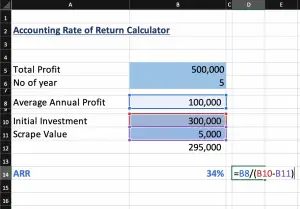# Accounting Rate of Return (ARR)

Accounting rate of return is the estimated accounting profit that the company makes from investment or the assets. It is the percentage of average annual profit over the initial investment cost. This method is very useful for project evaluation and decision making while the fund is limited. The company needs to decide whether or not to make a new investment such as purchasing an asset by comparing its cost and profit.

The company may accept a new investment if its ARR higher than a certain level, usually known as the hurdle rate which already approved by top management and shareholders. It aims to ensure that new projects will increase shareholders’ wealth for sustainable growth.

## Accounting Rate of Return Formula

$Accounting\ Rate\ of\ Return = {Average\ Annual\ Profit \over Initial\ Investment}$

Average Annual Profit is the total annual profit of the projects divided by the project terms, it is allowed to deduct the depreciation expense.

## Example: ARR for single project

Company A is considering investing in a new project which costs $500,000 and they expect to make a profit of$ 100,000 per year for 5 years.

ARR = 100,000 / 500,000 = 20%

Company ABC is planning to purchase new production equipment which cost $10M. The company expects to increase the revenue of$ 3M per year from this equipment, it also increases the operating expense of around $500,000 per year (exclude depreciation). The machinery has 10 years of useful life with zero scrap value. Please calculate the ARR. • Depreciation pe year =$ 10M / 10 year = $1M • Annual profit = ($ 3M –$0.5M –$ 1M) = $1.5M • ARR = 1.5 / 10 = 15% ## Example: simple rate of return method with salvage value Company P is considering to purchase new production equipment with the detail as below; • Cost of this equipment is$ 800,000
• It expects to use for 5 years with scrape value of 50,000 at the end of year 5
• The company expect to generate sale of 300,000 per year from it, however, it will require the maintaining cost of $30,000 per year Please calculate the ARR. Depreciation = ($ 800,000 – $30,000)/5year =$ 154,000 per year

Average annual profit = ($300,000 –$ 30,000 – $154,000) =$ 116,000

ARR = $116,000/$ 800,000 = 14.50 %

### Accounting Rate of Return in Excel

We can use Excel to help us in calculating ARR as following: